﻿ 基于非线性拟合投影寻踪模型和加速遗传算法通过Logistic回归分析一个地区供水能力

# 基于非线性拟合投影寻踪模型和加速遗传算法通过Logistic回归分析一个地区供水能力A Regional Water Supply Capacity Based on the Nonlinear Fitting of Projection Pursuit Model and Accelerating Genetic Algorithm by Logistic Regression Analysis

Abstract: This paper studies the problem of the sustainable development of regional water resources, the evaluation of the regional water supply ability; we used principal component analysis (PCA) to affect the physical and economic indicators of water filter, using a function model of calculating water supply capacity and projection pursuit model, the secondary fuzzy comprehensive evaluation model to assess the weights. This paper uses the logistic model to obtain water conditions in the region, and describes the impact that this situation has on the civic life in the region. This paper studies the index system of supply and demand of water resources as the main line, through the precise solution of the model; the region's ability of providing clean water levels was concluded, it also studied the water shortage, put forward the specific intervention plans to solve the problem of water shortage.

1. 前言

2. 基本方法

2.1. 通过主成分分析法筛选出影响区域最大供水量的主要指标 

${x}_{1},{x}_{2},\cdots ,{x}_{7}$ 分别表示：地下水储存量、地表径流、天然降水、水利工程、污水处理回用、海水淡化，水源污染。第i年 ${x}_{1},{x}_{2},\cdots ,{x}_{7}$ 的取值分别记为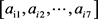，构造矩阵 $A={\left({a}_{ij}\right)}_{5×7}$

1) 对原始数据进行标准化处理。将各指标 ${a}_{ij}$ 转换成标准化指标 ${\stackrel{˜}{a}}_{ij}$ ，有：

${\stackrel{˜}{a}}_{ij}=\frac{{a}_{ij}-{\mu }_{j}}{{s}_{j}}\text{\hspace{0.17em}}\text{\hspace{0.17em}}\left(i=1,2,\cdots ,12;j=1,2,\cdots ,7\right)$ (2.1.1)

${\mu }_{j}=\frac{1}{12}\underset{i=1}{\overset{12}{\sum }}{a}_{ij};\text{\hspace{0.17em}}{s}_{j}=\sqrt{\frac{1}{12-1}}\sqrt{\underset{i=1}{\overset{12}{\sum }}{\left({a}_{ij}-{\mu }_{j}\right)}^{2}},\text{\hspace{0.17em}}\text{\hspace{0.17em}}j=1,2,\cdots ,7$ (2.1.2)

${\mu }_{j},{s}_{j}$ 为第j个指标的样本均值和样本标准差。

2) 计算相关系数矩阵R。相关系数矩阵 $R={\left({r}_{ij}\right)}_{7×7}$ ，有：

${r}_{ij}=\frac{\underset{k=1}{\overset{12}{\sum }}{\stackrel{˜}{a}}_{ki}\cdot {\stackrel{˜}{a}}_{kj}}{12-1},\text{\hspace{0.17em}}\text{\hspace{0.17em}}i,j=1,2,\cdots ,7$ (2.1.3)

3) 计算特征值和特征向量。计算相关系数矩阵R的特征值 ${\lambda }_{\text{1}}\ge {\lambda }_{\text{2}}\ge \cdots \ge {\lambda }_{\text{7}}\ge \text{0}$ ，及对应的标准化特征向量 ${\mu }_{\text{1}},{\mu }_{\text{2}},\cdots ,{\mu }_{7}$ ，其中 ${u}_{j}={\left[{u}_{1j},{u}_{2j},\cdots ,{u}_{7j}\right]}^{\text{T}}$ ，由特征向量组成7个新的指标变量

$\begin{array}{l}{y}_{1}={u}_{11}{\stackrel{˜}{x}}_{1}+{u}_{21}{\stackrel{˜}{x}}_{2}+\cdots +{u}_{71}{\stackrel{˜}{x}}_{7},\\ {y}_{2}={u}_{12}{\stackrel{˜}{x}}_{1}+{u}_{22}{\stackrel{˜}{x}}_{2}+\cdots +{u}_{72}{\stackrel{˜}{x}}_{7},\\ {y}_{5}={u}_{15}{\stackrel{˜}{x}}_{1}+{u}_{25}{\stackrel{˜}{x}}_{2}+\cdots +{u}_{77}{\stackrel{˜}{x}}_{7},\end{array}$ (2.1.4)

4) 选择 $p$ ( $p\le 7$ )个主成分，计算综合评价值。

1. 计算特征值 ${\lambda }_{j}\left(j=1,2,\cdots ,7\right)$ 的信息贡献率和累积贡献率。

${b}_{j}=\frac{{\lambda }_{j}}{\underset{k=1}{\overset{7}{\sum }}{\lambda }_{k}},\text{\hspace{0.17em}}j=1,2,\cdots ,7$ 为主成分 ${y}_{j}$ 的信息贡献率；

${\alpha }_{p}=\frac{\underset{k=1}{\overset{p}{\sum }}{\lambda }_{k}}{\underset{k=1}{\overset{7}{\sum }}{\lambda }_{k}}$ 为主成分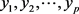的累积贡献率。

${\alpha }_{p}$ 接近于1( ${\alpha }_{p}=0.85,0.90,0.95$ )时，则选择前p个指标变量 ${y}_{1},{y}_{2},\cdots ,{y}_{5}$ 作为5个主成分，代替原来7个指标变量，从而可对5个主成分进行综合分析。

2. 计算综合得分：

$Z=\underset{j=1}{\overset{\text{p}}{\sum }}{b}_{j}{y}_{j}$ (2.1.5)

2.2. 用一次线性函数模型计算区域供水能力，并用模糊综合评价法评价， 最后提出投影寻踪模型

2.2.1. 一次线性函数模型计算供水能力Table 1. Results of principal component analysis

(1) 设X为该地区供水量，

(2) 设K为该地区总人口

(3) 设Y为该地区日平均供水量。

$Y=X/C$ (2.2.1)

2.2.2. 通过模糊综合评价方法评价现有一次线性函数模型

(一) 评价步骤

1. 取一级评价指标 = {水资源总量、污水排放总量、农业用水量、生活用水，再生水回用量}

2. 取评语集V = {影响很大 ${v}_{1}$ ，影响较大 ${v}_{2}$ ，影响一般大 ${v}_{3}$ ，影响较小 ${v}_{4}$ ，基本无影响 ${v}_{5}$ }

3. Delphi法确定权重

4. 确定模糊综合评判矩阵

5. 模糊综合评判

(二) 模型的求解

1. 一级指标权重的确定：

$A=\left[0.2,0.1,0.2,0.3,0.2\right]$

2. 二级指标权重为

${A}_{1}=\left[0.2,0.15,0.1,0.35,0.2\right]$

${A}_{2}=\left[0.25,0.15,0.2,0.2,0.3\right]$

${A}_{3}=\left[0.1,0.3,0.1,0.25,0.25\right]$

${A}_{4}=\left[0.15,0.25,0.1,0.35,0.15\right]$

${A}_{5}=\left[0.3,0.1,0.2,0.15,0.25\right]$

3. 对各个子因素集进行一级模糊综合评价得到

${B}_{1}={A}_{1}\cdot R=\left[0.28,0.29,0.06,0.37,0.01\right]$

${B}_{2}={A}_{2}\cdot R=\left[0.02,0.165,0.69,0.12,0.37\right]$

${B}_{3}={A}_{3}\cdot R=\left[0.25,0.33,0.125,0.26,0.35\right]$

${B}_{4}={A}_{4}\cdot R=\left[0.25,0.09,0.18,0.35,0.04\right]$

${B}_{5}={A}_{5}\cdot R=\left[0.28,0.14,0.02,0.34,0.07\right]$

$\begin{array}{c}C=A\cdot B=\left[0.2,0.1,0.2,0.3,0.2\right]\cdot \left[\begin{array}{ccccc}0.28& 0.29& 0.06& 0.37& 0.01\\ 0.02& 0.165& 0.69& 0.12& 0.37\\ 0.25& 0.33& 0.125& 0.26& 0.35\\ 0.25& 0.09& 0.18& 0.35& 0.04\\ 0.28& 0.14& 0.02& 0.34& 0.07\end{array}\right]\\ =\left[0.2769,0.0685,0.1026,0.1734,0.3481\right]\end{array}$

2.2.3. 投影寻踪模型 

1) 水资源利用率u1：现状75%频率的供水量/水资源总量，%

2) 缺水率u2：现状75% 频率的缺水量/供水量，%。

3) 灌溉率u3：灌溉面积/土地面积，%。

4) 降水量u4：多年平均降水量是区域水资源状况的自然禀赋，mm。

5) 人均占有水量u5：区域水资源总量/总人口，m3/人。

6) 径流深u6：地表径流/流域面积，mm。

7) 生态环境用水率u7：生态用水量/总水量，%。Table 2. Grading index of factors for comprehensive evaluation of regional water supply capacity

2.3. 回归模型 

1. 一个自变量的Logistic回归模型可写为：

$\text{Prob}\left(\text{event}\right)=\frac{1}{1+{\text{e}}^{-\left({b}_{0}+{b}_{1}x\right)}}$ (2.3.1)

$\text{Prob}\left(\text{event}\right)=\frac{1}{1+{\text{e}}^{-x}}$ (2.3.2)

$Z={b}_{0}+{b}_{1}{x}_{1}+{b}_{2}{x}_{2}+\cdots +{b}_{p}{x}_{p}$ (p为自变量的数量)(2.3.3)

${b}_{0},{b}_{1},\cdots ,{b}_{p}$ 分别为Logistic回归系数。

2. Logistic回归模型拟合度检验和系数检验 建立Logistic回归模型后，常用Hosmer-Losmer 统计量进行模型的拟合度检验，其表达式为

$\text{Chi-square}={\sum }_{1}^{n}{\left({x}_{s}-{x}_{y}\right)}^{2}/{x}_{y}$ (2.3.4)

$W={B}^{\prime }{V}^{-1}B$ (2.3.5)

2.4. 模型优缺点分析

2.4.1. 投影寻踪模型优缺点

2.4.2. 模糊综合评价模型的优缺点

2.4.3. Logistic回归分析预测法优缺点

Logistic回归分析预测法，是在分析现象自变量和因变量之间相关关系的基础上，建立变量之间的回归方程，并将回归方程作为预测模型，根据自变量在预测期的数量变化来预测因变量关系大多表现为相关关系，因此，回归分析预测法是一种重要的预测方法，当我们在对现象未来发展状况和水平进行预测时，如果能找到影响预测对象的主要因素，并且能够取得其数量资料，就可以采用回归分析预测法进行预测。它是一种具体的、行之有效的、实用价值很高的常用市场预测方法。

3. 说明区域缺水原因

3.1. 综合水资源开发情况选择北京为说明地区

3.2. 通过物理和经济短缺解释环境和社会驱动因素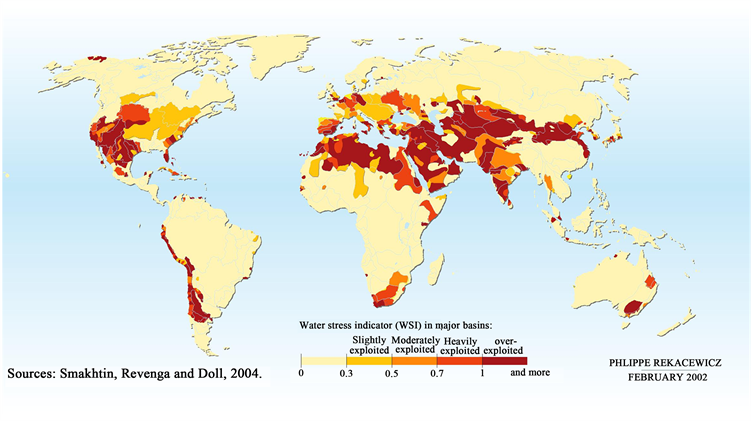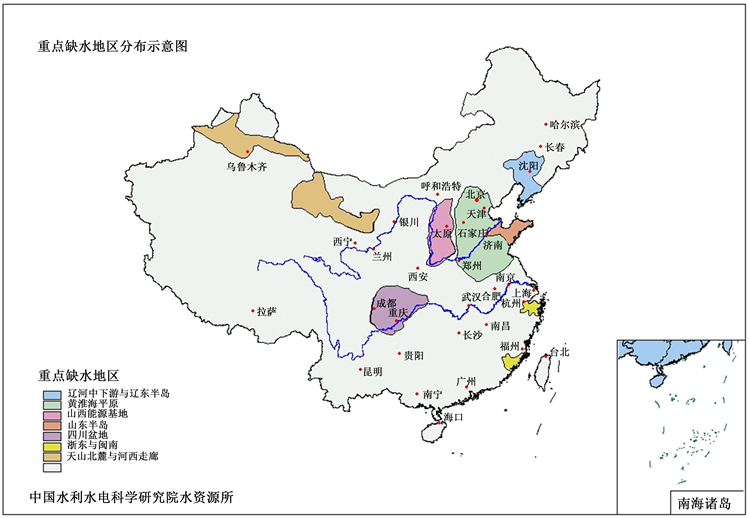Figure 2. Distribution map of water shortage areas in China (http://www.dljs.net/dltp/26365.html)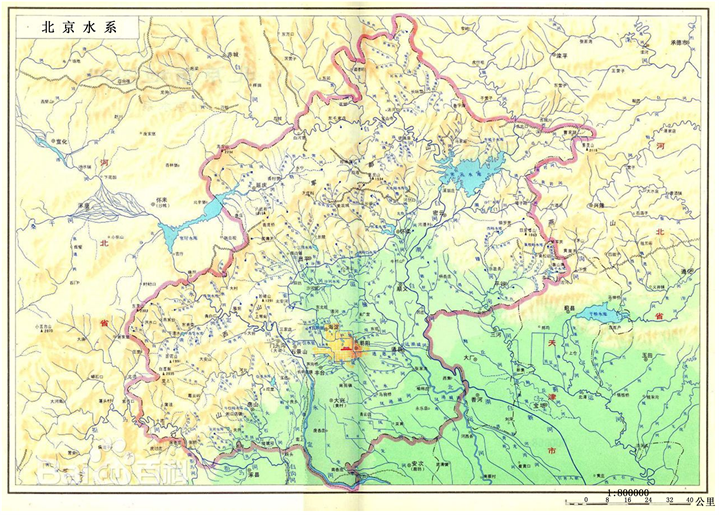Figure 3. Beijing river system (from Baidu Baike)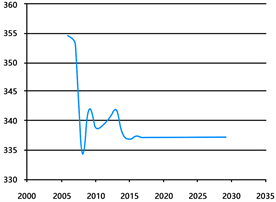Figure 4. Total agricultural using water (100 million cubic meters)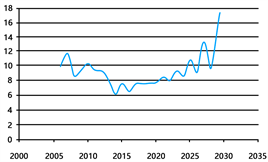Figure 5. EIW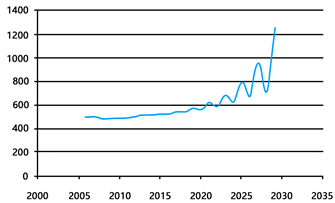Figure 6. Total water (100 million cubic meters)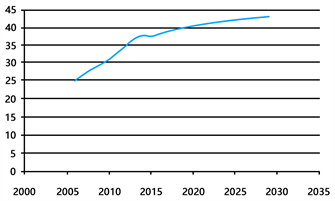Figure 7. Water supply capacity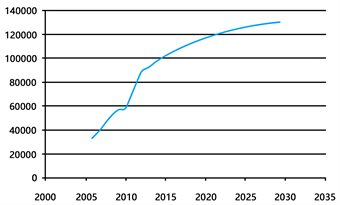Figure 8. Gross regional product (100 million yuan)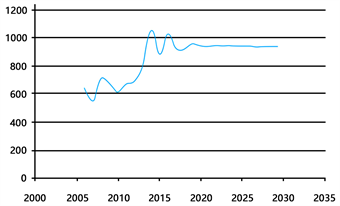Figure 9. Total resources (100 million cubic meters)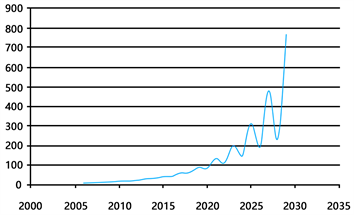Figure 10. Total using water (100 million cubic meters)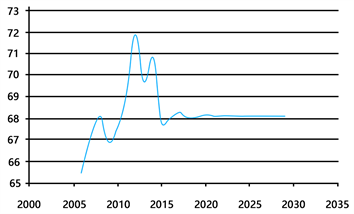Figure 11. Total using water (100 million cubic meters)

3.3. 用logistic模型展示北京未来15年水状况

 Hashimoto, T., Stedinger, J.R. and Loueks, D.P. (1982) Reliability, Resiliency and Vulnerability Criteria for Water Resources System per formance Evaluation. Water Resources Research, 18, 14-20. https://doi.org/10.1029/WR018i001p00014

 李建明, 谌黔鸣. n维空间中可连续变化的标准正交基的构造与投影追踪[J]. 贵州工学院学报, 1996(6): 24-28.

 裴丽萍. 水资源市场配置法律制度研究——一个以水资源利用为中心的水权制度构想[M]//环境资源法论丛第一卷, 北京: 法律出版社, 2001: 131.

 Bagel, M.S., Das Gupta, A. and Nayak, D.K. (2005) Amodelfor Optimal Allocati on of Water to Competing Demands. Water Resources Management, 19.

 National Agricultural Commission. Technical Specification for Land Use Survey. Publishing House of Surveying and Mapping, Beijing, 1984.

 Hashimoto, T., Stedinger, J.R. and Loueks, D.P. (1982) Reliability, Resiliency and Vulnerability Criteria for Water Resources System per formance Evaluation. Water Resources Research, 18, 14-20.
https://doi.org/10.1029/WR018i001p00014

 李建明, 谌黔鸣. n维空间中可连续变化的标准正交基的构造与投影追踪[J]. 贵州工学院学报, 1996(6): 24-28.

 裴丽萍. 水资源市场配置法律制度研究——一个以水资源利用为中心的水权制度构想[M]//环境资源法论丛第一卷, 北京: 法律出版社, 2001: 131.

 Bagel, M.S., Das Gupta, A. and Nayak, D.K. (2005) Amodelfor Optimal Allocati on of Water to Competing Demands. Water Resources Management, 19.

 National Agricultural Commission. Technical Specification for Land Use Survey. Publishing House of Surveying and Mapping, Beijing, 1984.

Top